# *-Autonomous category

Jump to: navigation, search

Letbe a symmetric closed monoidal category (cf. also Category). A functoris a duality functor if there exists an isomorphism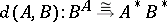, natural inand, such that for all objects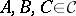the following diagram commutes:where in the bottom arrow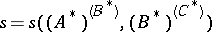.

A category is-autonomous if it is a symmetric monoidal closed category with a given duality functor.

It so happens that-autonomous categories have real-life applications: they are models of (at least the finite part of) linear logic [a2] and have uses in modelling processes.

An example of a-autonomous category is the category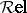of sets and relations; duality is given by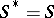. In fact,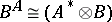.

From a given symmetric monoidal closed category and an object in it (that serves as a dualizing object) one can construct a-autonomous category (the so-called Chu construction, [a3]). It can be viewed as a kind of generalized topology.